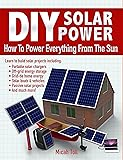# DIY Solar Power• Author: Micah Toll
• Full Title: DIY Solar Power
• Category: #books

### Highlights

• \$75/watt in the late 1970’s (Location 156)
• Voltage is a measure of electrical force, measured in the units of volts. In the water model, voltage is like the water pressure in the hose. (Location 229)
• Higher voltage is more force in the flow electricity, and higher current is a larger rate of the flow of electricity, as in the amount of total electricity flowing. (Location 270)
• Electrical power is easy to calculate. The power in watts is simply equal to the voltage in volts multiplied by the current in amps. So if I measure a voltage as 5 V and I measure the current flowing due to that voltage as 2 A, then the total electrical power in that circuit at the moment I measured is 10 W (5 V x 2 A = 10 W). But because power is an instantaneous measurement, that 10 W could easily change. If the current doubles to 4 A instead of 2 A, the new power in that circuit is now 20 W (5 V x 4 A = 20 W). (Location 278)
• solderless battery building kit (Location 1112)